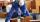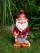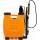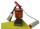Highway

Six trucks will be used to build the roadside 18 days. After 3 days, two more trucks were sent to build. How long will it take for material move?

Result

t =  14.25 d

Solution:Leave us a comment of this math problem and its solution (i.e. if it is still somewhat unclear...):Be the first to comment!To solve this verbal math problem are needed these knowledge from mathematics:

Do you have a linear equation or system of equations and looking for its solution? Or do you have quadratic equation?

Next similar math problems:

1. Construction of roadFor the construction of the road dam, it is supposed to transport six trucks a total of 18 days. After three days, two more cars were sent to the construction site. How long will it take to carry the material?
2. Working together on projectTwo employees working on the project. First make it alone for 20 days the second for 24 days. How many days will take to complete project if 5 days they work together then first will take two days holiday and after returning they completes a project toget
3. How oldThe student who asked how many years he answered: "After 10 years I will be twice as old than as I was four years ago. How old is student?
4. Hectoliters of waterThe pool has a total of 126 hectoliters of water. The first pump draws 2.1 liters of water per second. A second pump pumps 3.5 liters of water per second. How long will it take both pumps to drain four-fifths of the water at the same time?
5. Pumps A and BPump A fill the tank for 12 minutes, pump B for 24 minutes. How long will take to fill the tank if he is only three minutes works A and then both pumps A and B?
6. Bricklayers8 bricklayers build a house for 630 days. How many bricklayers have to add after 150 days, so then the whole building completed in (next) 320 days?
7. DwarfsFour dwarfs would prepare firewood for Snow White in580 minutes. After an hour and a half they recruit friends so that finished preparing the wood for 280 minutes. How many dwarfs they recruited?
8. Simple equationSolve for x: 3(x + 2) = x - 18
9. NovakNovak needed to dig up three of the same pit in the garden. The first pit dug father alone for 15 hours. His second dig son helped him and it did that in six hours. The third pit dug son himself. How long it took him?
10. SprayersThe vineyard can be sprayed first sprayer in 5 hours and with the second in 8 hours. How long would it take if we use sprayers both at once?
11. 1.5 divided1.5 divided by 1 = w divided by 4
12. QuizTested student answered correctly on a 4/ 8 questions. Wrong answers was 16. How many questions answered?
13. Three paintersOne painter would have painted the fence for 15 hours, the second in 12 hours. The plot had to be painted in four hours, so they called the third one, and all worked together. For what time would the third painter paint fence the fence alone?
14. Bed timeTiffany was 5 years old; her week night bedtime grew by ¼ hour each year. If, at age 18, her curfew time is 11pm, what was her bed time when she was 5 years old?
15. Three peopleThree people start doing a work at a same time. The first worked only 2 hours. The second ended 3 hours before the end. On an individual basis, it would take the first time to do the work 10 hours, second 12 hours and 15 hours third. How many hours did it.
16. TimeageSeven times of my age is 8 less than the largest two-digit number. How old I am?
17. PaintingJožko with Fero painted the bench for four hours. How many hours would take work if four friends help him?